# Crooks fluctuation theorem

The Crooks fluctuation theorem was developed by Gavin E. Crooks. It is also known as the Crooks Identity or the Crooks fluctuation relation. It is given by ( Eq. 2):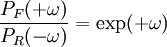$\frac{P_F(+\omega)}{P_R(-\omega)}= \exp({+ \omega})$

where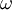$\omega$ is the entropy production,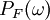$P_F(\omega)$ is the "forward" probability distribution of this entropy production, and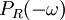$P_R(-\omega)$, time-reversed. This expression can be written in terms of work (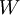$W$) (Eq. 11):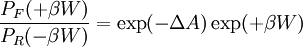$\frac{P_F(+\beta W)}{P_R(- \beta W)}= \exp (- \Delta A) \exp (+\beta W)$

where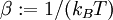$\beta := 1/(k_BT)$ where$k_B$ is the Boltzmann constant and$T$ is the temperature, and$A$ is the Helmholtz energy function.